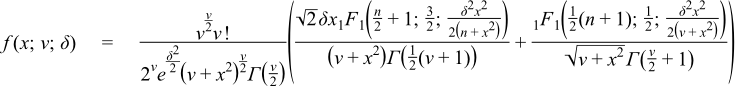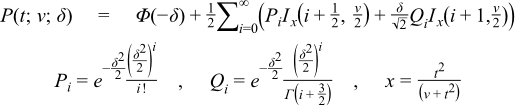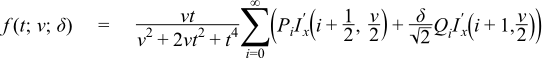#Boost C++ Libraries

...one of the most highly regarded and expertly designed C++ library projects in the world.

#### Noncentral T Distribution

`#include <boost/math/distributions/non_central_t.hpp>`
```namespace boost{ namespace math{

template <class RealType = double,
class Policy   = policies::policy<> >
class non_central_t_distribution;

typedef non_central_t_distribution<> non_central_t;

template <class RealType, class Policy>
class non_central_t_distribution
{
public:
typedef RealType  value_type;
typedef Policy    policy_type;

// Constructor:
non_central_t_distribution(RealType v, RealType delta);

// Accessor to degrees_of_freedom parameter v:
RealType degrees_of_freedom()const;

// Accessor to non-centrality parameter delta:
RealType non_centrality()const;
};

}} // namespaces
```

The noncentral T distribution is a generalization of the Students t Distribution. Let X have a normal distribution with mean δ and variance 1, and let ν S2 have a chi-squared distribution with degrees of freedom ν. Assume that X and S2 are independent. The distribution of tν(δ)=X/S is called a noncentral t distribution with degrees of freedom ν and noncentrality parameter δ.

This gives the following PDF:where 1F1(a;b;x) is a confluent hypergeometric function.

The following graph illustrates how the distribution changes for different values of ν and δ:##### Member Functions
```non_central_t_distribution(RealType v, RealType delta);
```

Constructs a non-central t distribution with degrees of freedom parameter v and non-centrality parameter delta.

Requires v > 0 (including positive infinity) and finite delta, otherwise calls domain_error.

```RealType degrees_of_freedom()const;
```

Returns the parameter v from which this object was constructed.

```RealType non_centrality()const;
```

Returns the non-centrality parameter delta from which this object was constructed.

##### Non-member Accessors

All the usual non-member accessor functions that are generic to all distributions are supported: Cumulative Distribution Function, Probability Density Function, Quantile, Hazard Function, Cumulative Hazard Function, mean, median, mode, variance, standard deviation, skewness, kurtosis, kurtosis_excess, range and support.

The domain of the random variable is [-∞, +∞].

##### Accuracy

The following table shows the peak errors (in units of epsilon) found on various platforms with various floating-point types. Unless otherwise specified, any floating-point type that is narrower than the one shown will have effectively zero error.

Table 2.6. Errors In CDF of the Noncentral T Distribution

Significand Size (bits)

Platform and Compiler

ν, δ < 600

53

Win32, Visual C++ 8

Peak=120 Mean=26

64

RedHat Linux IA32, gcc-4.1.1

Peak=121 Mean=26

64

Redhat Linux IA64, gcc-3.4.4

Peak=122 Mean=25

113

HPUX IA64, aCC A.06.06

Peak=115 Mean=24

CautionThe complexity of the current algorithm is dependent upon δ2: consequently the time taken to evaluate the CDF increases rapidly for δ > 500, likewise the accuracy decreases rapidly for very large δ.

Accuracy for the quantile and PDF functions should be broadly similar. The mode is determined numerically and cannot in principal be more accurate than the square root of floating-point type FPT epsilon, accessed using `boost::math::tools::epsilon<FPT>()`. For 64-bit `double`, epsilon is about 1e-16, so the fractional accuracy is limited to 1e-8.

##### Tests

There are two sets of tests of this distribution:

Basic sanity checks compare this implementation to the test values given in "Computing discrete mixtures of continuous distributions: noncentral chisquare, noncentral t and the distribution of the square of the sample multiple correlation coefficient." Denise Benton, K. Krishnamoorthy, Computational Statistics & Data Analysis 43 (2003) 249-267.

Accuracy checks use test data computed with this implementation and arbitary precision interval arithmetic: this test data is believed to be accurate to at least 50 decimal places.

The cases of large (or infinite) ν and/or large δ has received special treatment to avoid catastrophic loss of accuracy. New tests have been added to confirm the improvement achieved.

From Boost 1.52, degrees of freedom ν can be +∞ when the normal distribution located at δ (equivalent to the central Student's t distribution) is used in place for accuracy and speed.

##### Implementation

The CDF is computed using a modification of the method described in "Computing discrete mixtures of continuous distributions: noncentral chisquare, noncentral t and the distribution of the square of the sample multiple correlation coefficient." Denise Benton, K. Krishnamoorthy, Computational Statistics & Data Analysis 43 (2003) 249-267.

This uses the following formula for the CDF:Where Ix(a,b) is the incomplete beta function, and Φ(x) is the normal CDF at x.

Iteration starts at the largest of the Poisson weighting terms (at i = δ2 / 2) and then proceeds in both directions as per Benton and Krishnamoorthy's paper.

Alternatively, by considering what happens when t = ∞, we have x = 1, and therefore Ix(a,b) = 1 and:From this we can easily show that:and therefore we have a means to compute either the probability or its complement directly without the risk of cancellation error. The crossover criterion for choosing whether to calculate the CDF or its complement is the same as for the Noncentral Beta Distribution.

The PDF can be computed by a very similar method using:Where Ix'(a,b) is the derivative of the incomplete beta function.

For both the PDF and CDF we switch to approximating the distribution by a Student's t distribution centred on δ when ν is very large. The crossover location appears to be when δ/(4ν) < ε, this location was estimated by inspection of equation 2.6 in "A Comparison of Approximations To Percentiles of the Noncentral t-Distribution". H. Sahai and M. M. Ojeda, Revista Investigacion Operacional Vol 21, No 2, 2000, page 123.

Equation 2.6 is a Fisher-Cornish expansion by Eeden and Johnson. The second term includes the ratio δ/(4ν), so when this term become negligible, this and following terms can be ignored, leaving just Student's t distribution centred on δ.

This was also confirmed by experimental testing.

• "Some Approximations to the Percentage Points of the Noncentral t-Distribution". C. van Eeden. International Statistical Review, 29, 4-31.
• "Continuous Univariate Distributions". N.L. Johnson, S. Kotz and N. Balkrishnan. 1995. John Wiley and Sons New York.

The quantile is calculated via the usual derivative-free root-finding techniques, with the initial guess taken as the quantile of a normal approximation to the noncentral T.

There is no closed form for the mode, so this is computed via functional maximisation of the PDF.

The remaining functions (mean, variance etc) are implemented using the formulas given in Weisstein, Eric W. "Noncentral Student's t-Distribution." From MathWorld--A Wolfram Web Resource. http://mathworld.wolfram.com/NoncentralStudentst-Distribution.html and in the Mathematica documentation.

Some analytic properties of noncentral distributions (particularly unimodality, and monotonicity of their modes) are surveyed and summarized by:

Andrea van Aubel & Wolfgang Gawronski, Applied Mathematics and Computation, 141 (2003) 3-12.

 Copyright © 2006-2010, 2012, 2013 Paul A. Bristow, Christopher Kormanyos, Hubert Holin, Bruno Lalande, John Maddock, Johan Råde, Gautam Sewani, Benjamin Sobotta, Thijs van den Berg, Daryle Walker and Xiaogang Zhang Distributed under the Boost Software License, Version 1.0. (See accompanying file LICENSE_1_0.txt or copy at http://www.boost.org/LICENSE_1_0.txt)